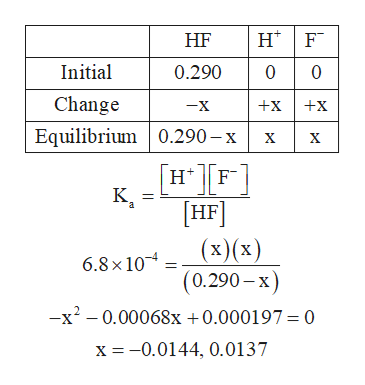Question
70 views

Find the pH and percent ionization of each HF solution. (Ka for HF is 6.8×10−4.)

a.  Find the pH of a 0.290 M HF solution.

b. Find the percent dissociation of a 0.290 M HF solution.

c. Find the pH of a 0.110 M HF solution.

d. Find the percent dissociation of a 0.110 M HF solution.

e. Find the pH of a 5.00×10−2 M HF solution.
f. Find the percent dissociation of a 5.00×10−2 M HF solution.

check_circle

Step 1

The pH of a solution is related to the hydrogen ion concentration of a solution. For a solution of weak acid, the hydrogen ion concentration is determined on the basis of its dissociation constant.

The pH of a solution is given by expression (1), in which, [H+] is the hydrogen ion concentration of the solution.

The percent dissociation of a species present in the solution is calculated by dividing the composition of the dissociated species (cd) by the composition of the undissociated species (cu), and then multiplying it with 100.

Step 2

a)

The amount of HF undergoes dissociation in the given solution is calculated by its dissociation constant expression and ICE table.help_outlineImage TranscriptioncloseH F HF 00 Initial 0.290 Change +x +x -X Equilibrium 0.290-x х х [н[F K HF (х)(x) (0.290-x) -x2-0.00068x + 0.000197 = 0 a 6.8x 10 x -0.0144, 0.0137 fullscreen
Step 3

The value of concentration of H+ and F- ions can never be negative. Therefore, the positive value 0.0137 wi...

### Want to see the full answer?

See Solution

#### Want to see this answer and more?

Solutions are written by subject experts who are available 24/7. Questions are typically answered within 1 hour.*

See Solution
*Response times may vary by subject and question.
Tagged in

### Equilibrium Concepts